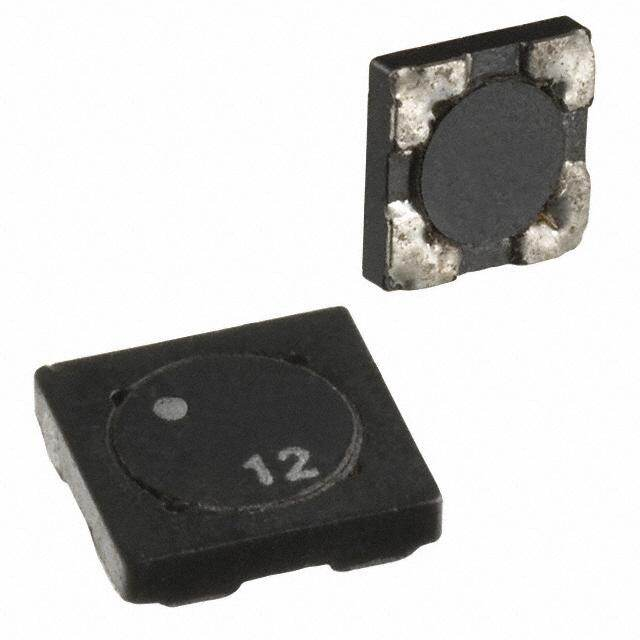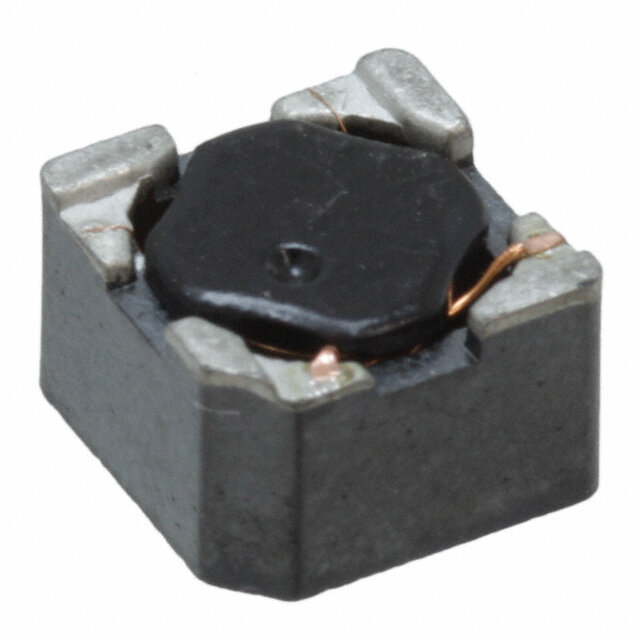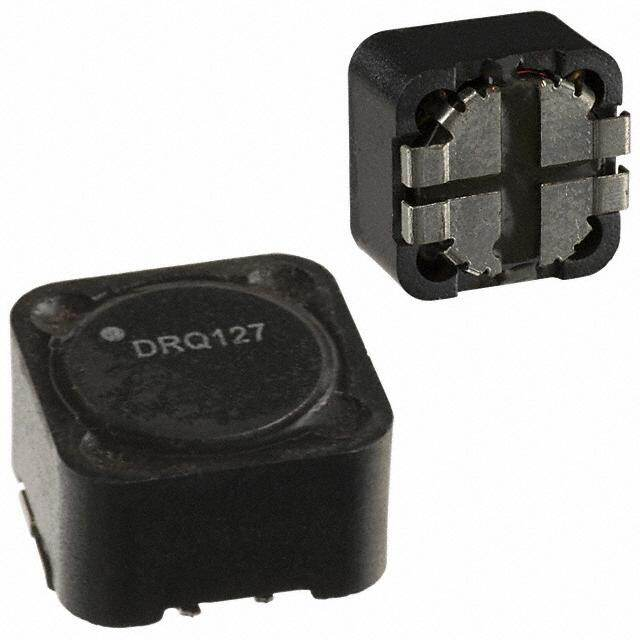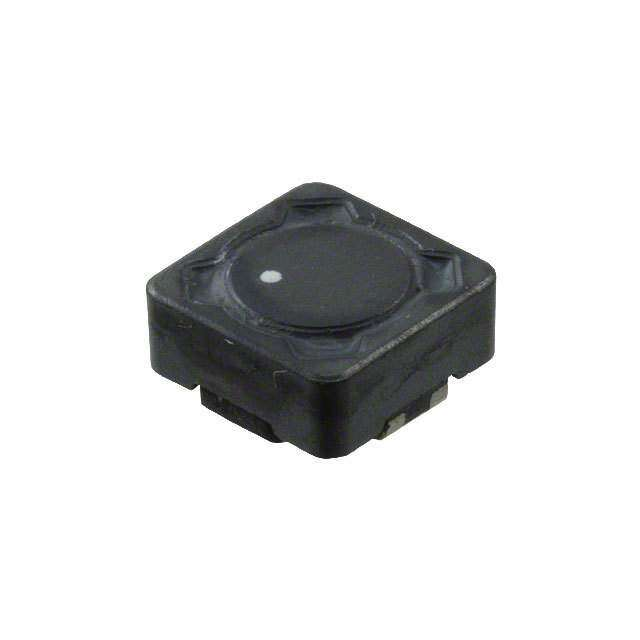RoHSx

1SDQ12-6R2-R Eaton - Electronics Division Y 屏蔽 2 Coil Inductor Array 25µH Inductance - Connected in Series 6.25µH Inductance - Connected in Parallel 238.7 毫欧 DC Resistance (DCR) - Parallel 1.02A 非标准
2SDQ12-1R0-R Eaton - Electronics Division Y 屏蔽 2 Coil Inductor Array 3.24µH Inductance - Connected in Series 810nH Inductance - Connected in Parallel 40.3 毫欧 DC Resistance (DCR) - Parallel 2.49A 非标准
3SDQ12-8R2-R Eaton - Electronics Division Y 屏蔽 2 Coil Inductor Array 33.64µH Inductance - Connected in Series 8.41µH Inductance - Connected in Parallel 331.8 毫欧 DC Resistance (DCR) - Parallel 868mA 非标准
4SDQ12-150-R Eaton - Electronics Division Y 屏蔽 2 Coil Inductor Array 60.84µH Inductance - Connected in Series 15.21µH Inductance - Connected in Parallel 576.6 毫欧 DC Resistance (DCR) - Parallel 658mA 非标准
5SDQ12-6R2-R Eaton - Electronics Division Y 屏蔽 2 Coil Inductor Array 25µH Inductance - Connected in Series 6.25µH Inductance - Connected in Parallel 238.7 毫欧 DC Resistance (DCR) - Parallel 1.02A 非标准
6SDQ12-8R2-R Eaton - Electronics Division Y 屏蔽 2 Coil Inductor Array 33.64µH Inductance - Connected in Series 8.41µH Inductance - Connected in Parallel 331.8 毫欧 DC Resistance (DCR) - Parallel 868mA 非标准
7SDQ12-150-R Eaton - Electronics Division Y 屏蔽 2 Coil Inductor Array 60.84µH Inductance - Connected in Series 15.21µH Inductance - Connected in Parallel 576.6 毫欧 DC Resistance (DCR) - Parallel 658mA 非标准
8SDQ12-1R0-R Eaton - Electronics Division Y 屏蔽 2 Coil Inductor Array 3.24µH Inductance - Connected in Series 810nH Inductance - Connected in Parallel 40.3 毫欧 DC Resistance (DCR) - Parallel 2.49A 非标准
9SDQ12-150-R Eaton - Electronics Division Y 屏蔽 2 Coil Inductor Array 60.84µH Inductance - Connected in Series 15.21µH Inductance - Connected in Parallel 576.6 毫欧 DC Resistance (DCR) - Parallel 658mA 非标准
10SDQ12-6R2-R Eaton - Electronics Division Y 屏蔽 2 Coil Inductor Array 25µH Inductance - Connected in Series 6.25µH Inductance - Connected in Parallel 238.7 毫欧 DC Resistance (DCR) - Parallel 1.02A 非标准
11SDQ12-1R0-R Eaton - Electronics Division Y 屏蔽 2 Coil Inductor Array 3.24µH Inductance - Connected in Series 810nH Inductance - Connected in Parallel 40.3 毫欧 DC Resistance (DCR) - Parallel 2.49A 非标准
12SDQ12-8R2-R Eaton - Electronics Division Y 屏蔽 2 Coil Inductor Array 33.64µH Inductance - Connected in Series 8.41µH Inductance - Connected in Parallel 331.8 毫欧 DC Resistance (DCR) - Parallel 868mA 非标准
1374488540070 Wurth Electronics Inc. Y 无屏蔽 2 Coil Inductor Array Inductance - Connected in Series Inductance - Connected in Parallel DC Resistance (DCR) - Parallel 1.9A 非标准
1474488540070 Wurth Electronics Inc. Y 无屏蔽 2 Coil Inductor Array Inductance - Connected in Series Inductance - Connected in Parallel DC Resistance (DCR) - Parallel 1.9A 非标准
1574488540070 Wurth Electronics Inc. Y 无屏蔽 2 Coil Inductor Array Inductance - Connected in Series Inductance - Connected in Parallel DC Resistance (DCR) - Parallel 1.9A 非标准
16 暂无 DRQ127-2R2-G Eaton - Electronics Division 屏蔽 2 Coil Inductor Array 8.108µH Inductance - Connected in Series 2.027µH Inductance - Connected in Parallel 3.73 毫欧 DC Resistance (DCR) - Parallel 12.5A 非标准
17DRQ127-2R2-R Eaton - Electronics Division Y 屏蔽 2 Coil Inductor Array 8.108µH Inductance - Connected in Series 2.027µH Inductance - Connected in Parallel 3.73 毫欧 DC Resistance (DCR) - Parallel 12.5A 非标准
18DRQ127-2R2-R Eaton - Electronics Division Y 屏蔽 2 Coil Inductor Array 8.108µH Inductance - Connected in Series 2.027µH Inductance - Connected in Parallel 3.73 毫欧 DC Resistance (DCR) - Parallel 12.5A 非标准
19DRQ127-2R2-R Eaton - Electronics Division Y 屏蔽 2 Coil Inductor Array 8.108µH Inductance - Connected in Series 2.027µH Inductance - Connected in Parallel 3.73 毫欧 DC Resistance (DCR) - Parallel 12.5A 非标准
20744878004 Wurth Electronics Inc. Y 屏蔽 2 Coil Inductor Array 18.8µH Inductance - Connected in Series 4.7µH Inductance - Connected in Parallel 75 毫欧最大 DC Resistance (DCR) - Parallel 1.3A 非标准

### 还有更多产品分类没有全部展示，请重新设置过滤条件筛选

手机、邮箱任意一项必填，公司、联系人选填